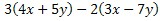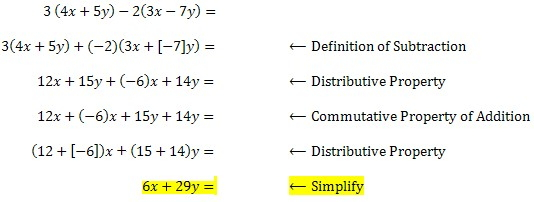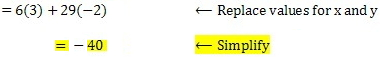Algebraic Expressions

Algebraic Expressions

Numeric expressions apply operations to numbers. For example, 2(3 + 8) is a numeric expression.  Algebraic expressions include at least one variable and at least one operation (addition, subtraction, multiplication, division).  For example, 2(x + 8y) is an algebraic expression.

Example

Simplify the algebraic expression:Then evaluate the simplified expression for x = 3 and y = -2.

Solution

To solve, simplify the algebraic expression using the basic properties of real numbersNow replace x with 3 and y with -2 in the simplified expression.